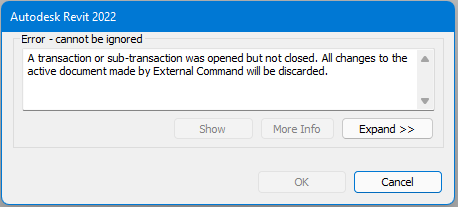# Transaction error

Hi,

I cant figure out why revit is giving me a transaction error on the following.I only have one transaction at the end of my code. (if i remove any reference to revit or autodesk and just print the variables instead of trying to update the parameters within revit the code works as intended.

Basically what the code is doing is grabbing an address out of a file. Grabbing the suburb and looking up a postcode and state and adding it to the text and then trying to insert it into the project address field within revit.

``````from Autodesk.Revit.DB import *
import csv
import time
import os
import re

keywords = ['Unit', 'Lot', 'Villa', 'No.']
suburb = False

#this gets the appdata location from the OS
appdata = os.getenv('APPDATA')

doc = __revit__.ActiveUIDocument.Document

project_info = FilteredElementCollector(doc).OfCategory(BuiltInCategory.OST_ProjectInformation).ToElements()

file = open(appdata + "\LGX\programSettings.txt")

#this imports all the lines in the file to a variable

project_number = project_data_import

file.close()

multi_suburb = True
else:
multi_suburb = False
else:

if i.strip():

if multi_suburb == True:
suburb_check = multi_word_suburb_check
else:

try:
except ValueError:
number_check = ''

line_4 = []
line_1 = ''
line_2 = ''
line_3 = ''
suburb_check = suburb_check.upper().rstrip('\n')

if row == suburb_check:
line_4.append(row)
line_4.append(row)
line_3 = suburb_check
line_4 = ', '.join(line_4)
suburb_word_count = len(suburb_check.split(' '))
suburb = True
for x in range(suburb_word_count):
if suburb == False:
line_4 = ''

result = []

for i in keywords:
if result != []:
line_1 = ' '.join(address[min(result):max(result) + 2])
if multi_suburb == True:
else:
elif isinstance(number_check, int):
line_1 = 'No. ' + line_1
elif suburb == True:
else:

address = str(line_1.upper() + "\n" + line_2.upper() + '\n' + line_3.upper() + '\n' + line_4.upper())

t = Transaction(doc, 'Fill in titleblock')
t.Start()
project_info.Number = project_number
t.Commit()

``````

I’m going to take a guess and say that whatever you’re trying to do within the transaction is not working?
Maybe run a simplified copy of the script just to be sure that the 2 lines of code within the transaction are working first?

Thanks for your help. I tried hardcoding a string and it still didnt work.
looks like i was referencing the wrong elements to change.
I changed the second line below. It is now updating the parameters.

``````doc = __revit__.ActiveUIDocument.Document

project_info = doc.ProjectInformation
``````
2 Likes

I’m not 99% sure, but this variable is a list. That’s why it’s not working. You need an instance of ProjectInformation, so probably sth like this :

``````t = Transaction(doc, 'Fill in titleblock')
t.Start()
project_info.Number = project_number